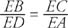## Segments of Chords Secants Tangents

In Figure 1, chords QS and RT intersect at P. By drawing QT and RS, it can be proven that Δ QPT ∼ Δ RPS. Because the ratios of corresponding sides of similar triangles are equal, ac = db. The Cross Products Property produces ( a) ( b) = ( c) ( d). This is stated as a theorem.Figure 1 Two chords intersecting inside a circle.

Theorem 83: If two chords intersect inside a circle, then the product of the segments of one chord equals the product of the segments of the other chord.

Example 1: Find x in each of the following figures in Figure 2.Figure 2 Two chords intersecting inside a circle.

In Figure 3, secant segments AB and CD intersect outside the circle at E. By drawing BC and AO, it can be proven that Δ EBC ∼ Δ EDA. This makesFigure 3 Two secant segments intersecting outside a circle.

By using the Cross‐Products Property,

• (EB)(EA) = (ED)(EC)

This is stated as a theorem.

Theorem 84: If two secant segments intersect outside a circle, then the product of the secant segment with its external portion equals the product of the other secant segment with its external portion.

Example 2: Find x in each of the following figures in 4.Figure 4 More secant segments intersecting outside a circle.

In Figure 5, tangent segment AB and secant segment BD intersect outside the circle at B. By drawing AC and AD, it can be proven that Δ ADB ∼ Δ CAB. Therefore,Figure 5 A tangent segment and a secant segment intersecting outside a circle.This is stated as a theorem.

Theorem 85: If a tangent segment and a secant segment intersect outside a circle, then the square of the measure of the tangent segment equals the product of the measures of the secant segment and its external portion.

Also,

Theorem 86: If two tangent segments intersect outside a circle, then the tangent segments have equal measures.

Example 3: Find x in the following figures in 6.Figure 6 A tangent segment and a secant segment (or another tangent segment) intersecting outside a circle.